# The hedging hyperprior for the exponential distribution

## 2023-02-28

suppressMessages(suppressWarnings(library(MASS)))
suppressMessages(suppressWarnings(library(tidyverse)))
knitr::opts_chunk$set(echo=TRUE, fig.width=8, fig.height=2) I want to write a paper about the hedging hyperprior. I need to work out some simple examples first. Here is an example involving the exponential distribution. ### A simple Baysian analysis with an informative prior. Consider a Bayesian analysis of an outcome variable that has an exponential distribution. The density of the exponential distribution is $$f(x) = \theta^{-1} e^{-x / \theta}$$ You have an informative prior on $$\theta$$ that has an inverse gamma distribution with prior parameters $$\alpha_0$$ and $$\beta_0$$. $$g(\theta; \alpha_0, \beta_0) = \frac {\beta^\alpha_0} {\Gamma(\alpha_0)} \theta^{-\alpha_0-1} e^{-\beta_0 / \theta}$$ The mean of this prior distribution is $$\frac{\beta}{\alpha_0-1}$$ You collect a sample of n data points, $$\mathbf{X} = X_1, X_2, ..., X_n$$. This gives you a likelihood of $$L(\mathbf{X}) = \prod_{i=1}^n f(X_i) = \theta^{-n} e^{-n \bar X / \theta}$$ and a posterior distribution of $$h(\theta|\mathbf{X}) \propto \frac {\beta_0^{\alpha_0-1}} {\Gamma(\alpha_0)} \theta^{-\alpha_0-1} e^{-\beta_0 / \theta} \theta^{-n} e^{-n \bar X / \theta}$$ which simplifies to $$h(\theta | \mathbf{X}) \propto \theta^{-\alpha_0-1-n} e^{-(\beta_0 + n\bar X) / \theta}$$ which, thanks to the miracle of conjugancy, is an inverse gamma distribution. With posterior paramaters $$\alpha_1 = \alpha_0 + n$$ $$\beta_1 = \beta_0 + n\bar X$$ The posterior mean $$\frac{\beta_0 + n\bar X}{\alpha_0-1+n}$$ can be written as a weighted average of the prior mean and the mean of the observed data. $$\Big( \frac {\alpha_0-1} {\alpha_0-1+n}\Big) \frac {\beta_0} {\alpha-1} + \Big( \frac {n} {\alpha-1+n}\Big) \bar X$$ ### A simple numerical example x_max <- 3 a0 <- 41 b0 <- 44 n <- 60 xbar <- 2.1 a1 <- a0 + n b1 <- b0 + n*xbar posterior_alpha <- a0+n posterior_beta <- b0+n*xbar x_grid <- seq(0.01, x_max, length=1000) f <- function(x, theta) { theta^(-1) * exp(-x/theta) } g <- function(theta, alpha, beta) { beta^alpha/gamma(alpha) * theta^(-alpha-1) * exp(-beta/theta) } prior05 <- 1/qgamma(c(0.975), a0, b0) prior95 <- 1/qgamma(c(0.025), a0, b0) post05 <- 1/qgamma(c(0.975), a1, b1) post95 <- 1/qgamma(c(0.025), a1, b1)  Let’s illustrate this with an informative prior with $$\alpha$$ = 41 and $$\beta$$ = 44. g_grid <- g(x_grid, a0, b0) outside <- x_grid < prior05 | x_grid > prior95 x_color <- ifelse(outside, "white", "gray") data.frame(x=x_grid, y=g_grid) %>% ggplot(aes(x, y)) + geom_segment(aes(xend=x, yend=0), color=x_color) + geom_line() + xlab(" ") + ylab(" ")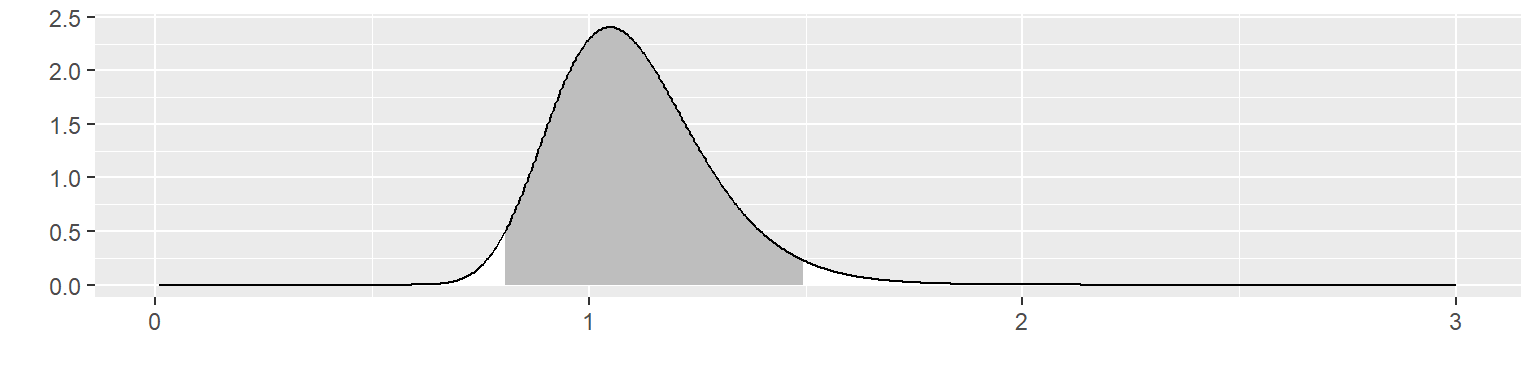This corresponds to a fairly informative prior distribution. This distribution places $$\theta$$ within 0.81 and 1.50 with 95% probability. Let’s see what happens with this informative prior if you observe 60 values of x with a mean of 2.1. The likelihood is f_grid <- f(xbar, x_grid)^n x_color <- "white" data.frame(x=x_grid, y=f_grid) %>% ggplot(aes(x, y)) + geom_line() + xlab(" ") + ylab(" ")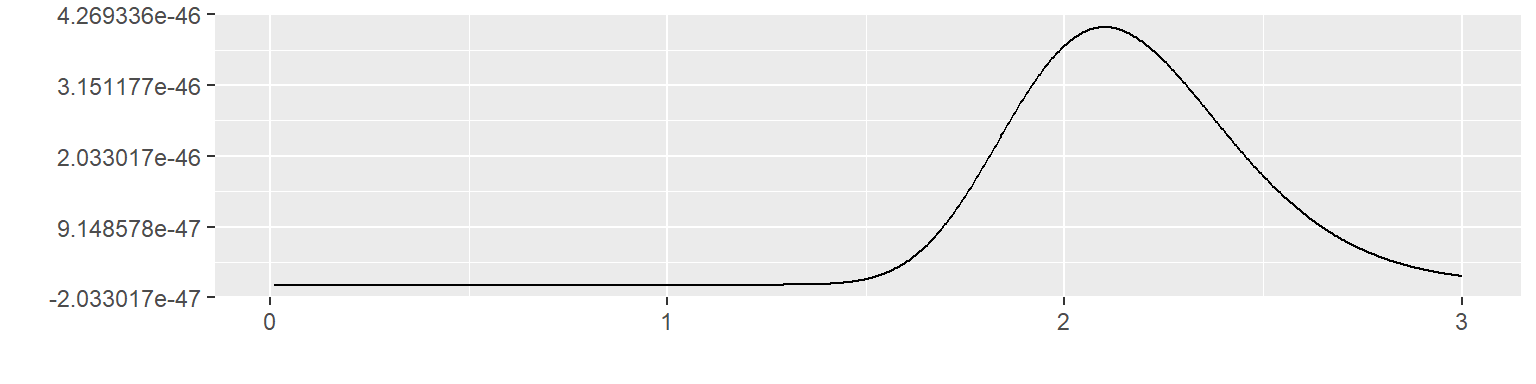Multiply these two together to get the posterior distribution. h_grid <- g(x_grid, a1, b1) outside <- x_grid < post05 | x_grid > post95 x_color <- ifelse(outside, "white", "gray") data.frame(x=x_grid, y=h_grid) %>% ggplot(aes(x, y)) + geom_segment(aes(xend=x_grid, yend=0), color=x_color) + geom_line() + xlab(" ") + ylab(" ")The posterior distribution is not close to either the prior distribution or the likelihood. It places $$\theta$$ within 1.40 and 2.07 with 95% probability. It excludes the prior mean of 1.10 and it excludes 2.1, the mean of the data. ### The hedging hyperprior When the likelihood of your data and your informative prior are in conflict, you may wish to downweight the informative prior. You can do this by including a hedging hyperprior. Define your prior distribution as an inverse gamma distributions with parameters $$1+\lambda (\alpha-1)$$ and $$\lambda \beta$$ where $$\lambda$$ ranges between 0 and 1. Then place a hyperprior on the parameter $$\lambda$$. The uniform(0,1) distribution is a reasonable choice for the hyperprior, but others are possible. knitr::opts_chunk$set(echo=TRUE, fig.width=8, fig.height=8)
lambda_grid <- seq(0, 1, length=60)
theta_grid <- seq(0, x_max, length=60)

gstar <- function(theta, lambda) {g(theta, lambda*(a0-1)+1, lambda*(b0-1)+1)}
density <- outer(theta_grid, lambda_grid, gstar)
op <- par(bg = "white")
z_max <- max(density, na.rm=TRUE)
persp(
theta_grid,
lambda_grid,
density,
zlim=c(0, 1.1*z_max),
xlab="theta",
ylab="lambda",
theta = 135,
phi = 30,
expand=0.5,
ticktype="detailed")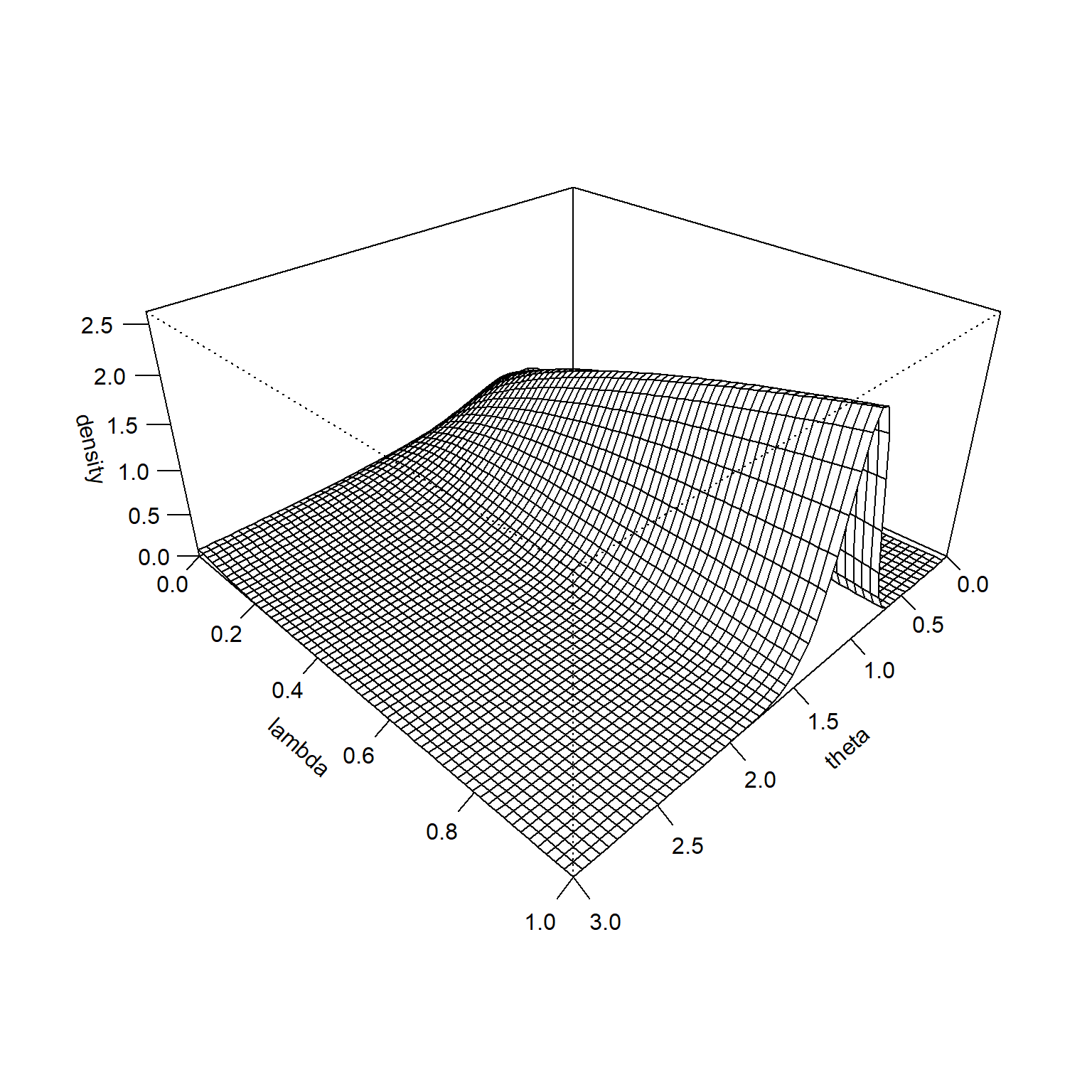It is hard to see at this scale, but the prior distribution is higher for small values of $$\lambda$$ when $$\theta$$ is very large. This becomes more obvious if you remove the middle of the surface and rescale the Z-axis. There is a similar pattern on the far side of the graph. The prior trends towards a higher prior for small values of $$\lambda$$ when $$\theta$$ is very small.

This illustrates how the hedging hyperprior does its magic. It puts more probability on large values of $$\lambda$$ (gives closer to full weight for the informative prior) when you observe data values close to the prior mean. It puts more probability on small values of $$\lambda$$ (gives much less weight to the informative prior) when the data values are much larger or much smaller than the prior mean.

density <- outer(theta_grid, lambda_grid, gstar)
# density[12:32, ] <- NA
# density[11:40, ] <- NA
density[density>0.3] <- 0.3
op <- par(bg = "white")
z_max <- max(density, na.rm=TRUE)
persp(
theta_grid,
lambda_grid,
density,
zlim=c(0, 1.1*z_max),
xlab="theta",
ylab="lambda",
theta = 135,
phi = 30,
expand=0.5,
ticktype="detailed")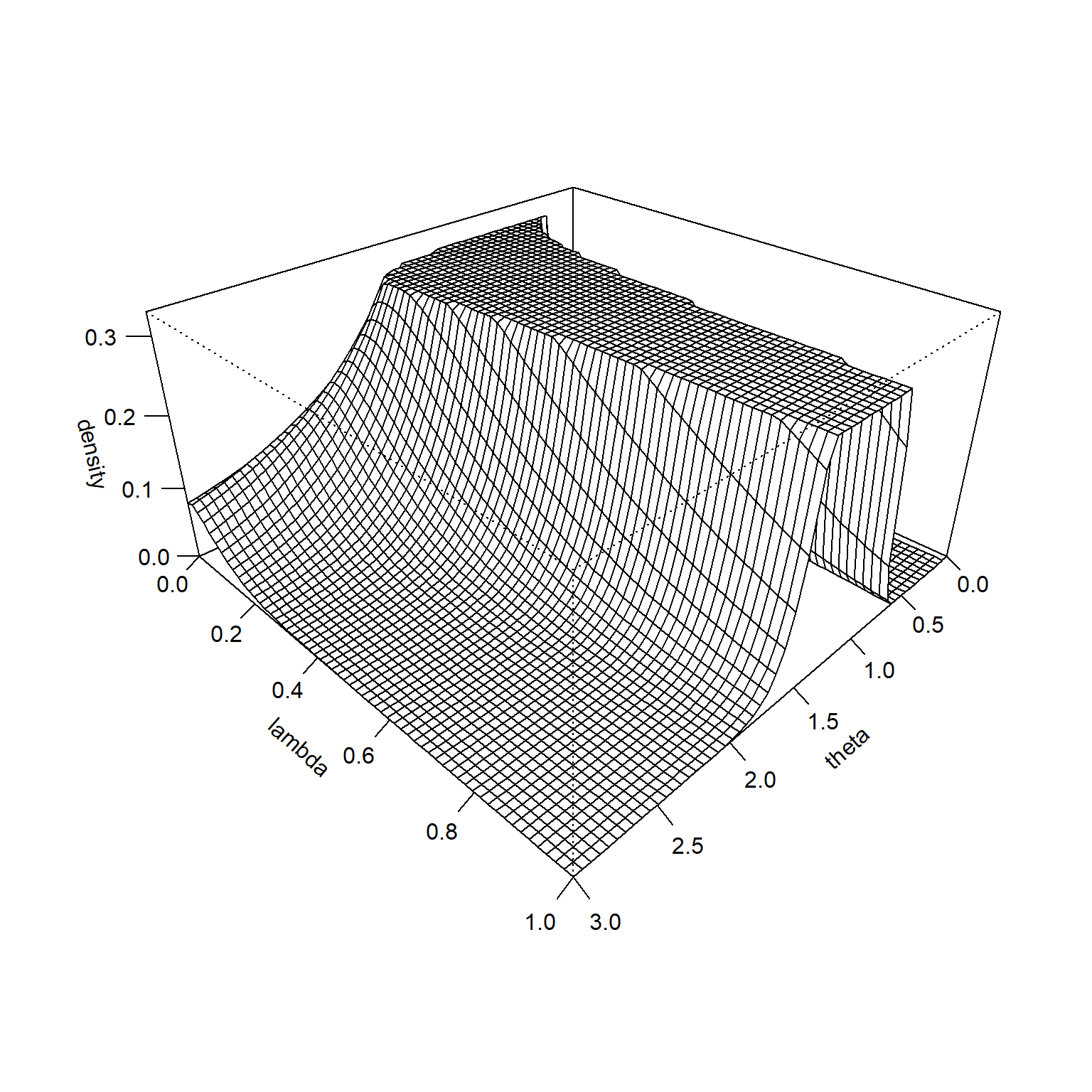The likelihood function is constant across all values of $$\lambda$$, so its surface looks like the following.

lambda_grid <- seq(0, 1, length=60)
theta_grid <- seq(0, x_max, length=60)

fstar <- function(theta, lambda) {f(xbar, theta)^n}
likelihood <- outer(theta_grid, lambda_grid, fstar)
z_max <- max(likelihood, na.rm=TRUE)
persp(
theta_grid,
lambda_grid,
likelihood,
zlim=c(0, 1.1*z_max),
xlab="theta",
ylab="lambda",
theta = 135,
phi = 30,
expand=0.5,
ticktype="detailed")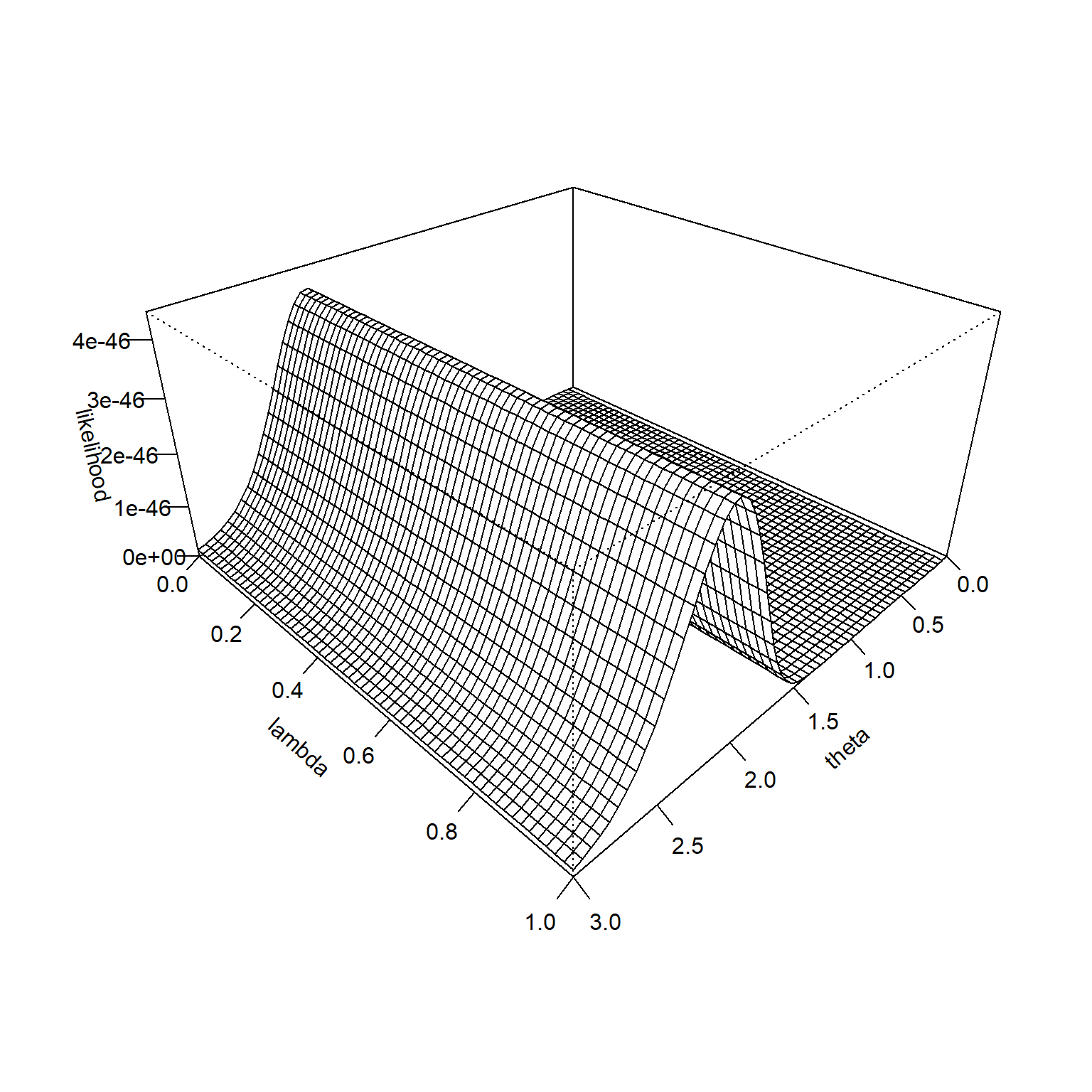The product of the prior and the likelihood is proportional to the posterior distribution.

lambda_grid <- seq(0, 1, length=60)
theta_grid <- seq(0, x_max, length=60)

hstar <- function(theta, lambda) {g(theta, lambda*(a0-1)+1, lambda*(b0-1)+1)*f(xbar, theta)^n}
density <- outer(theta_grid, lambda_grid, hstar)
z_max <- max(density, na.rm=TRUE)
persp(
theta_grid,
lambda_grid,
density,
zlim=c(0, 1.1*z_max),
xlab="theta",
ylab="lambda",
theta = 135,
phi = 30,
expand=0.5,
ticktype="detailed")A contour plot shows where the probability is concentrated. The labels on the contour lines represent how much probabilty is found inside the contour line.

density <- density / sum(density, na.rm=TRUE)
density %>%
as.vector %>%
sort %>%
rev -> sorted_density
cumulative_p <- 0
for (p in sorted_density) {
cumulative_p <- p + cumulative_p
density[density==p] <- cumulative_p
}
contour(
theta_grid,
lambda_grid,
density,
labcex=1,
levels=c(10, 25, 50, 75, 90)/100,
xlab="theta",
ylab="lambda")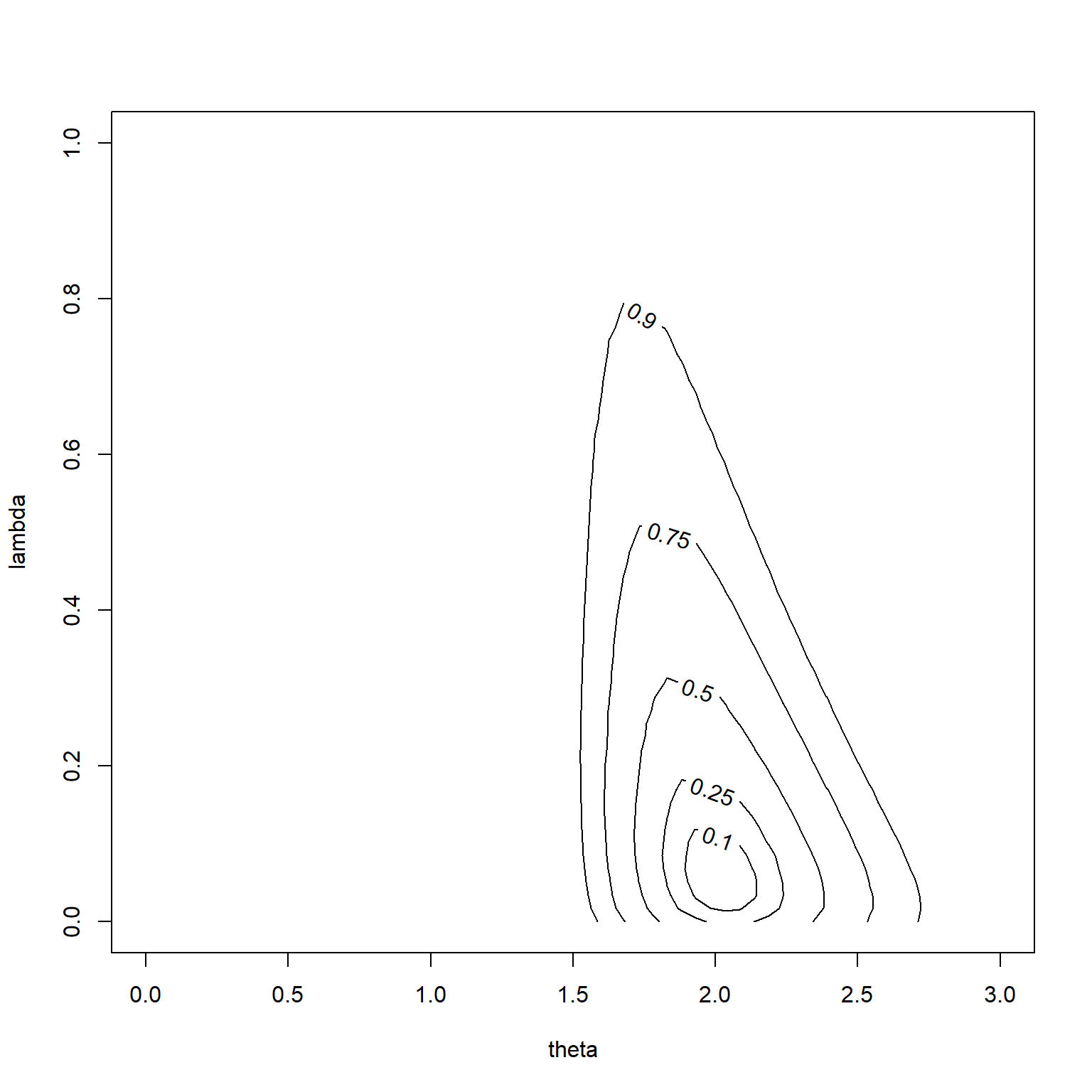You can see that the hedging hyperprior did its job. The most probable values of $$\lambda$$ are small and the prior mean (1.1) is largely ignored in favor of the mean of the data (2.1).